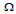# Digital Electronics - Multivibrators and 555 Timer - Discussion

### Discussion :: Multivibrators and 555 Timer - General Questions (Q.No.31)

31.

A 0.3-F capacitor has an initial charge of 2.7 V. If it is discharged through a 10-kresistor for 6.5 ms, how much voltage will be left across the capacitor?

 [A]. 0.0 V [B]. 0.309 V [C]. 2.7 V [D]. 2.295 V

Explanation:

No answer description available for this question.

 Priya said: (Dec 2, 2011) The voltage formula is given as Vc = V(1 - e-t/RC) which equals: Vc = 2.7(1-e-.0065/.003) [RC = .003 seconds from above] Therefore, Vc = 2.39 volts voltage will be left across the capacitor is 2.7V-2.39V=0.31V

 Anjana said: (Nov 14, 2016) Correct @Priya.